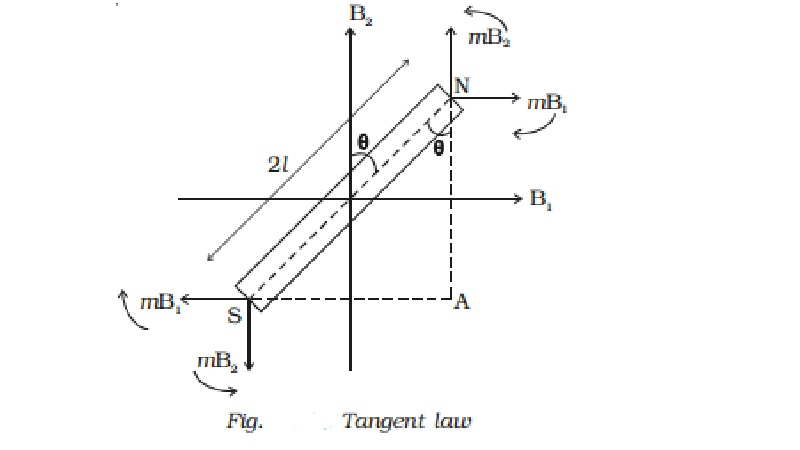Home | | Physics | Tangent law

# Tangent lawA magnetic needle suspended, at a point where there are two crossed magnetic fields acting at right angles to each other, will come to rest in the direction of the resultant of the two fields.

Tangent law

A magnetic needle suspended, at a point where there are two crossed magnetic fields acting at right angles to each other, will come to rest in the direction of the resultant of the two fields.

B1 and B2 are two uniform magnetic fields acting at right angles to each other. A magnetic needle

placed in these two fields will be subjected to two torques tending to rotate the magnet in opposite

directions. The torque τ1 due to the two equal and opposite parallel forces mB1 and mB1 tend to set the magnet parallel to B1. Similarly the torque τ2 due to the two equal and opposite parallel forces mB2 and mB2 tends to set the magnet parallel to B2. In a position where the torques balance each other, the magnet comes to rest. Now the magnet makes an angle ? with B2 as shown in the Fig.The deflecting torque due to the forces mB1 and mB1

τ1 = mB1 ? NA

=  mB1 ? NS cos θ

=  mB1 ? 2l cos θ

=  2l mB1 cos θ

τ 1 = MB1cos θ

Similarly the restoring torque due to the forces mB2 and mB2

τ 2 = mB2 ? SA

=  mB2 ? 2l sin θ

=  2lm ? B2 sin θ

=  τ 2 = MB2 sin θ

At equillibrium,

τ 1 = τ 2

MB1 cos θ = MB2 sin θ

B1 = B2 tan θ

This is called Tangent law

Invariably, in the applications of tangent law, the restoring magnetic field B2 is the horizontal component of Earth?s magnetic field Bh.

Study Material, Lecturing Notes, Assignment, Reference, Wiki description explanation, brief detail
11th 12th std standard Class Physics sciense Higher secondary school College Notes : Tangent law |